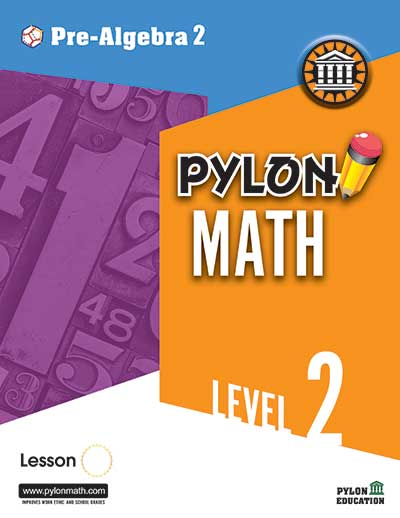# PreAlgebra 21. ### Equations

• One-step equations
• Two-step equations
• Combined operations
• Two variable terms
• Variables on both sides
• Inequalities
2. ### Word Problems

• Numerical expressions
• Algebraic Expressions
• Writing equations
3. ### Percent

• Percent proportions
• Percent of a number
• Percent of change
• Finding original numbers
4. ### Interest

• Simple interest
• Amount to be repaid
• Finding length of time
• Finding rates
• Compound interest
5. ### The Coordinate Planes

• Solutions of equations
• Graphing equations
• Changing equations for y
• Slopes of equations
6. ### The Coordinate Planes

• The slope-intercept forms
• Graphing a line with slope
and y-intercept
• Parallel, perpendicular,
and intersecting lines
x-and y-intercepts
7. ### Box-and-Whisker Plots

• Median, Mode, and Mean
• Quartiles, Ranges
• Box-and Whisker Plots
• Limits, Outliers
1. ### Probability

• Counting Principle
• With Replacement, Without Replacement
• With Repeat, Without Repeat
• Permutations, Combinations
2. ### Mixed Review

• Probability, Equations, Word Problems
3. ### Areas

• Rectangles, Complex Figures
• Parallelograms, Triangles
• Trapezoids
• Combined Areas
4. ### Circles

• Circumferences, Arcs
• Perimeters of Complex Figures
• Areas of Circles
• Areas of Complex Figures
5. ### Volumes

• Rectangular Prisms, Triangular Prisms
• Trapezoidal Prisms, Cylinders
• Solids, Pyramids, Cones, Spheres
• Hemispheres
6. ### Surface Areas

• Rectangular Prisms, Triangular Prisms
• Trapezoidal Prisms, Lateral Areas
• Cylinders, Cones
• Spheres and Hemispheres

### Special Right Triangles

• 45’ Right Triangles
• 30’ - 60’ Right Triangles

• Mixed Review